# Use your handouts, notes, returned quiz, and warm-ups for example problems on em spectrum, average atomic mass, isotopes, and mole conversions Unit 2 practice test Multiple Choice

 Date 18.04.2018 Size 79.78 Kb. #41337
Topics Covered on the unit 2 Test:

Modern atomic theory – this includes the 4 scientists we studied, their experiments, and the conclusions of these experiments

Isotopes/Atoms

Average atomic mass

Mole conversions

Electromagnetic Spectrum – conceptual understanding of EM spectrum and frequency/wavelength energy problems
*** Use your handouts, notes, returned quiz, and warm-ups for example problems on EM spectrum, average atomic mass, isotopes, and mole conversions

Unit 2 practice test
Multiple Choice

Identify the choice that best completes the statement or answers the question.
____ 1. According to Dalton's atomic theory, atoms
 a. are destroyed in chemical reactions. b. can be divided. c. of each element are identical in size, mass, and other properties. d. of different elements cannot combine.

____ 2. Which of the following statements is true?

 a. Atoms of the same element may have different masses. b. Atoms may be divided in ordinary chemical reactions. c. Atoms can never combine with any other atoms. d. Matter is composed of large particles called atoms.

____ 3. The rays produced in a cathode tube in early experiments were

 a. unaffected by a magnetic field. c. found to carry a positive charge. b. deflected away from a negative plate. d. striking the cathode.

____ 4. The discovery of the electron resulted from experiments using

 a. gold foil. c. neutrons. b. cathode rays. d. alpha particles.

____ 5. Who discovered the nucleus by bombarding gold foil with positively charged particles and noting that some particles were widely deflected?

 a. Rutherford c. Chadwick b. Dalton d. Bohr

____ 6. Rutherford's experiments led to the discovery of the

 a. electron. c. nucleus. b. cathode ray. d. neutron.

____ 7. The mass of a neutron is

 a. about the same as that of a proton. c. double that of a proton. b. about the same as that of an electron. d. double that of an electron.

____ 8. The charge on the electron cloud

 a. prevents compounds from forming. b. balances the charge on the nucleus. c. attracts electron clouds in other atoms to form compounds. d. does not exist.

____ 9. Isotopes are atoms of the same element that have different

 a. principal chemical properties. c. numbers of protons. b. masses. d. numbers of electrons.

____ 10. The atomic number of oxygen, 8, indicates that there are eight

 a. protons in the nucleus of an oxygen atom. b. oxygen nuclides. c. neutrons outside the oxygen atom's nucleus. d. energy levels in the oxygen atom's nucleus.

____ 11. The average atomic mass of an element is the average of the atomic masses of its

 a. naturally occurring isotopes. c. nonradioactive isotopes. b. two most abundant isotopes. d. artificial isotopes.

____ 12. The average atomic mass of an element

 a. is the mass of the most abundant isotope. b. may not equal the mass of any of its isotopes. c. cannot be calculated. d. always adds up to 100.

____ 13. Zn-66 (atomic number 30) has

 a. 30 neutrons. c. 36 neutrons. b. 33 neutrons. d. 96 neutrons.

____ 14. Phosphorus-33 (atomic number 15) contains

 a. 33 protons. c. 33 neutrons. b. 18 neutrons. d. 18 protons.

____ 15. The number of atoms in 1 mol of carbon is

 a. 6.022  1022. c. 5.022  1022. b. 6.022  1023. d. 5.022  1023.

____ 16. Molar mass

 a. is the mass in grams of one mole of a substance. b. is numerically equal to the average atomic mass of the element. c. both a and b d. neither a nor b

____ 17. The mass of two moles of oxygen atoms (atomic mass 16 amu) is

 a. 16 g. c. 48 g. b. 32 g. d. 64 g.

____ 18. A prospector finds 39.39 g of gold (atomic mass 196.9665 amu). She has

 a. 1.20  1023 atoms. c. 4.30  1023 atoms. b. 2.30  1023 atoms. d. 6.02  1023 atoms.

____ 19. The speed of an electromagnetic wave is equal to the product of its wavelength and its

 a. mass. c. velocity. b. color. d. frequency.

____ 20. Planck's constant

 a. depends on the frequency of the radiation. b. depends on the mass of the radiation. c. depends on the wavelength of the radiation. d. is the same for all forms of radiation.

____ 21. Bohr's theory helped explain why

 a. electrons have negative charge. b. most of the mass of the atom is in the nucleus. c. excited hydrogen gas gives off certain colors of light. d. atoms combine to form molecules.

22. How has Dalton's theory about atomic particles been modified by modern science?
23. What is the relationship between isotopes, mass number, and neutrons?
24. Why do chemists work with moles instead of individual atoms?
Problem
25. How many atoms are present in 80.0 mol of zirconium?
26. How many moles of platinum are equivalent to 1.20  1024 atoms?
27. How many moles of iron are equivalent to 1.11  1025 atoms?
28. Determine the mass in grams of 5.00 mol of oxygen. The molar mass of oxygen is 16.00 g/mol.
29. Determine the mass in grams of 10.0 mol of bromine. The molar mass of bromine is 79.90 g/mol.
30. Calculate the number of atoms in 10.0 g of sulfur (molar mass 32.07 g/mol).
Essay
31. Why do scientists use Dalton's theory, even though parts of it have been proven wrong? How much of Dalton's theory do scientists still accept?
32. What can you determine about the atomic structure of an element and one of its isotopes if you know the atomic number and mass numbers?
33. Explain the significance of Avogadro's constant, 6.022  1023. What is the relationship between it and the molar mass of oxygen, 16.00 g/mol?
Unit 2 practice test

MULTIPLE CHOICE
1. ANS: C PTS: 1
2. ANS: A PTS: 1
3. ANS: B PTS: 1
4. ANS: B PTS: 1
5. ANS: A PTS: 1
6. ANS: C PTS: 1
7. ANS: A PTS: 1
8. ANS: B PTS: 1
9. ANS: B PTS: 1
10. ANS: A PTS: 1
11. ANS: A PTS: 1
12. ANS: B PTS: 1
13. ANS: C PTS: 1
14. ANS: B PTS: 1
15. ANS: B PTS: 1
16. ANS: C PTS: 1
17. ANS: B PTS: 1
18. ANS: A PTS: 1
19. ANS: D PTS: 1
20. ANS: D PTS: 1
21. ANS: C PTS: 1
22. ANS:

The discovery of subatomic particles proved that atoms are divisible. Dalton believed that atoms were indivisible.

PTS: 1
23. ANS:

Isotopes are atoms of the same element with different numbers of neutrons and therefore different mass numbers.

PTS: 1
24. ANS:

A mole is a collection of atoms that is large enough to measure in the laboratory. A single atom is too small.

PTS: 1
PROBLEM
25. ANS: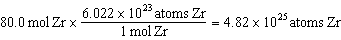4.82  1025 atoms Zr

PTS: 1
26. ANS: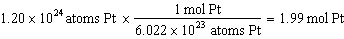1.99 mol Pt

PTS: 1
27. ANS: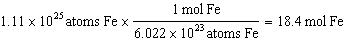18.4 mol Fe

PTS: 1
28. ANS: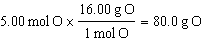80.0 g O

PTS: 1
29. ANS: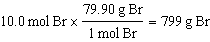799 g Br

PTS: 1
30. ANS: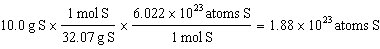1.88  1023 atoms S

PTS: 1
ESSAY

31. ANS:

Dalton's theory led to the modern theory of the atom. Although scientists now know that atoms can be divided, most of his early theory is still accepted.

PTS: 1
32. ANS:

The atomic number equals the number of protons in the nucleus of an atom and also equals the number of electrons in the neutral atom. The mass number is the sum of the number of protons and neutrons and can be used, with the atomic number, to find the number of neutrons. An isotope of an element has a different mass number but the same atomic number as the element.

PTS: 1
33. ANS:

Avogadro's constant is the number of particles in 1 mol of a substance. Because the molar mass of oxygen atoms is 16.00 g/mol, the mass of 1 mol, or 6.022  1023, oxygen atoms is 16.00 g.

PTS: 1